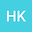A Computational Approach for the Classifications of 8-dimensional Nilsolitons
•In this study, we present an algorithm in MATLAB to classify $8$ dimensional non-abelian nilsoliton metric Lie algebras with singular Gram matrices. With this algorithm, we can compute Lie brackets, structure constants, index, rank and eigenvalues of the nilsoliton derivation. This paper can be considered as a follow up paper to our previous study \cite{Kad3}. In our previous paper, we defined an algorithm that helps to classify algebras that admit simple derivations and singular Gram matrices $U$. Since the Gram matrices are singular, there exists infinitely many solutions to $Uv=_m$, where the solutions are exactly the structure constants’ squares. In order the algebra to be a Lie algebra, the structure constants has to satisfy the Jacobi identity. In our previous work, we did not present a methodology to classify algebras that satisfy Jacobi identity. But in this paper, we extend the capability in such a way that we are able to create and solve the Jacobi identity/identities with the help of computer algorithms for each index set. Therefore we completely classify all indecomposible nilsolitons in dimension $8$. Several examples are provided for the illustration of the methodology.